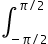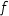Maths-
General
Easy

Question

# Let f : R →R and g : R →R be continuous functions, then the value of(f(x) + f(-x)) (g(x) – g(-x)) dx, is equal to

## The correct answer is: 0

###A functionis odd if f(x)=f(x)f(x)=f(x)#### With Turito Foundation.#### Get an Expert Advice From Turito.